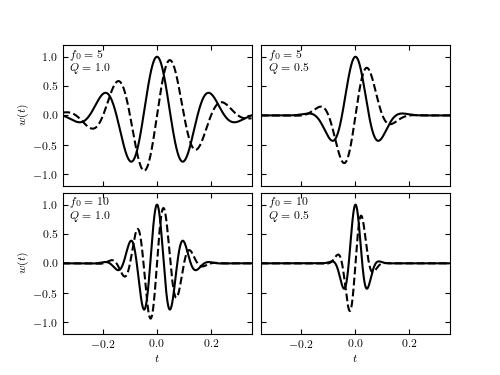# Examples of Wavelets¶

Figure 10.9

Wavelets for several values of wavelet parameters Q and f0. Solid lines show the real part and dashed lines show the imaginary part (see eq. 10.16).```
```
```# Author: Jake VanderPlas
#   The figure produced by this code is published in the textbook
#   "Statistics, Data Mining, and Machine Learning in Astronomy" (2013)
#   To report a bug or issue, use the following forum:
import numpy as np
from matplotlib import pyplot as plt

from astroML.fourier import FT_continuous, IFT_continuous, sinegauss

#----------------------------------------------------------------------
# This function adjusts matplotlib settings for a uniform feel in the textbook.
# Note that with usetex=True, fonts are rendered with LaTeX.  This may
# result in an error if LaTeX is not installed on your system.  In that case,
# you can set usetex to False.
if "setup_text_plots" not in globals():
from astroML.plotting import setup_text_plots
setup_text_plots(fontsize=8, usetex=True)

#------------------------------------------------------------
# Set up the wavelets
t0 = 0
t = np.linspace(-0.4, 0.4, 10000)
f0 = np.array([5, 5, 10, 10])
Q = np.array([1, 0.5, 1, 0.5])

# compute wavelets all at once
W = sinegauss(t, t0, f0[:, None], Q[:, None])

#------------------------------------------------------------
# Plot the wavelets
fig = plt.figure(figsize=(5, 3.75))

# in each panel, plot and label a different wavelet
for i in range(4):
ax.plot(t, W[i].real, '-k')
ax.plot(t, W[i].imag, '--k')

ax.text(0.04, 0.95, "\$f_0 = %i\$\n\$Q = %.1f\$" % (f0[i], Q[i]),
ha='left', va='top', transform=ax.transAxes)

ax.set_ylim(-1.2, 1.2)
ax.set_xlim(-0.35, 0.35)

ax.xaxis.set_major_locator(plt.MultipleLocator(0.2))

if i in (0, 1):
ax.xaxis.set_major_formatter(plt.NullFormatter())
else:
ax.set_xlabel('\$t\$')

if i in (1, 3):
ax.yaxis.set_major_formatter(plt.NullFormatter())
else:
ax.set_ylabel('\$w(t)\$')

plt.show()
```